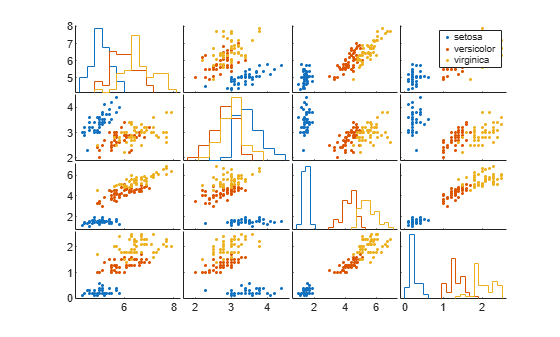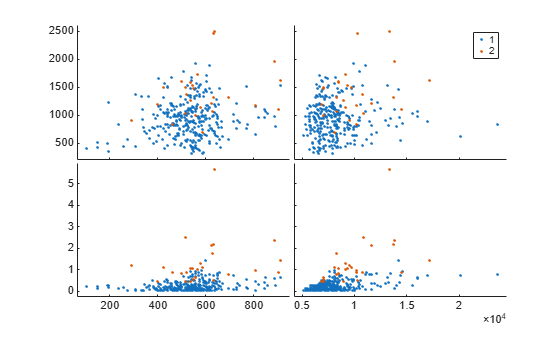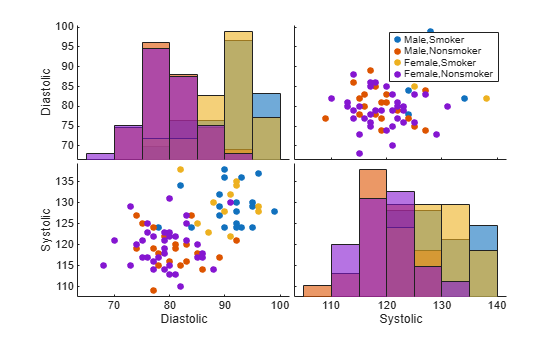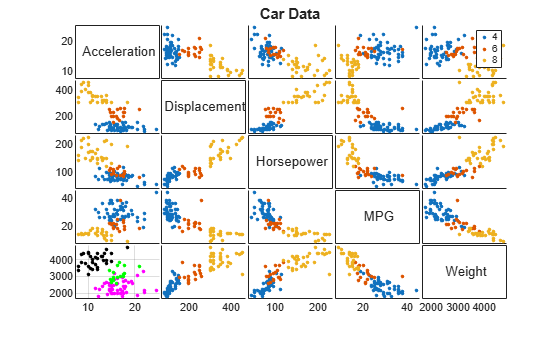# gplotmatrix

Matrix of scatter plots by group

## Syntax

``gplotmatrix(X,[],group)``
``gplotmatrix(X,Y,group)``
``gplotmatrix(X,Y,group,clr,sym,siz)``
``gplotmatrix(X,Y,group,clr,sym,siz,doleg)``
``gplotmatrix(X,[],group,clr,sym,siz,doleg,dispopt)``
``gplotmatrix(X,[],group,clr,sym,siz,doleg,dispopt,xnam)``
``gplotmatrix(X,Y,group,clr,sym,siz,doleg,[],xnam,ynam)``
``gplotmatrix(parent,___)``
``[h,ax,bigax] = gplotmatrix(___)``

## Description

example

````gplotmatrix(X,[],group)` creates a matrix of scatter plots and histograms of the data in `X`, grouped by the grouping variable in `group`. Each off-diagonal plot in the resulting figure is a scatter plot of a column of `X` against another column of `X`. The software also plots the outlines of the grouped histograms in the diagonal plots of the plot matrix. `X` and `group` must have the same number of rows.```

example

````gplotmatrix(X,Y,group)` creates a matrix of scatter plots. Each plot in the resulting figure is a scatter plot of a column of `X` against a column of `Y`. For example, if `X` has p columns and `Y` has q columns, then the figure contains a q-by-p matrix of scatter plots. All plots are grouped by the grouping variable `group`. The input arguments `X`, `Y`, and `group` must all have the same number of rows.```
````gplotmatrix(X,Y,group,clr,sym,siz)` specifies the marker color `clr`, symbol `sym`, and size `siz` for each group.```
````gplotmatrix(X,Y,group,clr,sym,siz,doleg)` controls whether a legend is displayed in the figure. `gplotmatrix` creates a legend by default.```
````gplotmatrix(X,[],group,clr,sym,siz,doleg,dispopt)` controls the display options for the diagonal plots in the plot matrix of `X`.```

example

````gplotmatrix(X,[],group,clr,sym,siz,doleg,dispopt,xnam)` labels the x-axes and y-axes of the scatter plots using the column names specified in `xnam`. The input argument `xnam` must contain one name for each column of `X`. Set `dispopt` to `'variable'` to display the variable names along the diagonal of the scatter plot matrix.```

example

````gplotmatrix(X,Y,group,clr,sym,siz,doleg,[],xnam,ynam)` labels the x-axes and y-axes of the scatter plots using the column names specified in `xnam` and `ynam`. The input arguments `xnam` and `ynam` must contain one name for each column of `X` and `Y`, respectively.```
````gplotmatrix(parent,___)` creates the scatter plot matrix in the figure or panel specified by `parent`. Specify `parent` as the first input argument followed by any of the input argument combinations in the previous syntaxes.```

example

````[h,ax,bigax] = gplotmatrix(___)` returns graphics handles to the individual plots and the entire scatter plot matrix.You can pass in `[]` for `clr`, `sym`, `siz`, `doleg`, and `dispopt` to use their default values.```

## Examples

collapse all

Create a matrix of scatter plots for each combination of variables in a data set. Group the data according to a separate variable.

Load the `fisheriris` data set, which contains flower data. The four columns of `meas` are the sepal length, sepal width, petal length, and petal width of the flowers. `species` contains the flower species names: setosa, versicolor, and virginica. Visually compare the flower measurements across flower species.

```load fisheriris gplotmatrix(meas,[],species)```In the matrix of scatter plots, the x-axis of the leftmost column of scatter plots corresponds to sepal length, the first column in `meas`. Similarly, the y-axis of the bottom row of scatter plots corresponds to petal width, the last column in `meas`. Therefore, the scatter plot in the bottom left of the matrix compares sepal length values (along the x-axis) to petal width values (along the y-axis). The color of each point depends on the species of the flower.

The diagonal plots are histograms rather than scatter plots. For example, the plot in the top left of the matrix shows the distribution of sepal length values for each species of flower.

Create scatter plots comparing a subset of the variables in a data set to another subset of variables. Group the data according to a separate variable.

Load the `discrim` data set.

`load discrim`

The `ratings` array contains rating values of 329 US cities for the nine categories listed in the `categories` array. The `group` array contains a city size code that is equal to `2` for the 26 largest cities, and `1` otherwise.

Create a matrix of scatter plots to compare the first two categories, `climate` and `housing`, with the fourth and seventh categories, `crime` and `arts`. Specify `group` as the grouping variable to visually distinguish the data for large and small cities.

```X = ratings(:,1:2); Y = ratings(:,[4 7]); gplotmatrix(X,Y,group)```The matrix of scatter plots shows the specified comparisons, with each city size group represented by a different color.

Adjust the appearance of the plots by specifying marker colors and symbols, and labeling the axes with the rating categories.

```xnames = categories(1:2,:); ynames = categories([4 7],:); gplotmatrix(X,Y,group,'br','.o',[],'on',[],xnames,ynames)```Create a matrix of scatter plots comparing data variables by using two grouping variables.

Load the `patients` data set. Compare patient diastolic and systolic blood pressure values. Group the patients according to their gender and smoker status. Convert `Smoker` to a categorical variable to have more descriptive labels in the legend. Display grouped histograms along the diagonal of the plot matrix by using the `'grpbars'` display option, and label the axes.

```load patients X = [Diastolic Systolic]; labeledSmoker = categorical(Smoker,[true false],{'Smoker','Nonsmoker'}); group = {Gender,labeledSmoker}; color = lines(4)```
```color = 4×3 0 0.4470 0.7410 0.8500 0.3250 0.0980 0.9290 0.6940 0.1250 0.4940 0.1840 0.5560 ```
```xnames = {'Diastolic','Systolic'}; gplotmatrix(X,[],group,color,[],[],[],'grpbars',xnames)```For example, the scatter plot in the bottom left of the matrix shows that smokers (blue and yellow markers) tend to have higher diastolic and systolic blood pressure values, regardless of gender.

Create a matrix of scatter plots that display grouped data. Modify the appearance of one of the scatter plots.

Load the `carsmall` data set. Create a scatter plot matrix using different car measurements. Group the cars by the number of cylinders. Specify the group colors, and display the car variable names along the diagonal of the plot matrix. Add a title to the plot matrix.

```load carsmall X = [Acceleration Displacement Horsepower MPG Weight]; color = lines(3)```
```color = 3×3 0 0.4470 0.7410 0.8500 0.3250 0.0980 0.9290 0.6940 0.1250 ```
```xnames = {'Acceleration','Displacement','Horsepower','MPG','Weight'}; [h,ax] = gplotmatrix(X,[],Cylinders,color,[],[],[],'variable',xnames); title('Car Data')```Change the appearance of the scatter plot in the bottom left of the matrix by using `h` and `ax`. First, change the colors of the data points in the scatter plot. Then, add grid lines to the scatter plot.

```bottomleftPlot = h(5,1,:); bottomleftPlot(1).Color = 'blue'; bottomleftPlot(2).Color = 'red'; bottomleftPlot(3).Color = 'yellow'; bottomleftAxes = ax(5,1); bottomleftAxes.XGrid = 'on'; bottomleftAxes.YGrid = 'on';```## Input Arguments

collapse all

Input data, specified as an n-by-p numeric matrix, datetime array, or duration array. `gplotmatrix` creates a matrix of plots using the columns of `X`. If you do not specify an additional input matrix `Y`, then `gplotmatrix` creates a p-by-p matrix of plots. The off-diagonal plots are scatter plots, and the diagonal plots depend on the value of `dispopt`. In each scatter plot, `gplotmatrix` plots one column of `X` against another column of `X`. The points in the scatter plots are grouped according to `group`.

If you specify `Y`, then `gplotmatrix` creates a q-by-p matrix of scatter plots using the p columns of `X` and the q columns of `Y`.

Data Types: `single` | `double` | `datetime` | `duration`

Input data, specified as an n-by-q numeric matrix, datetime array, or duration array. `gplotmatrix` creates a q-by-p matrix of scatter plots using the p columns of `X` and the q columns of `Y`. For each column of the plot matrix, the x-axis values of the scatter plots are the same as the values in the corresponding column of `X`. Similarly, for each row of the plot matrix, the y-axis values of the scatter plots are the same as the values in the corresponding column of `Y`. The points in the scatter plots are grouped according to `group`.

`X` and `Y` must have the same number of rows.

Data Types: `single` | `double` | `datetime` | `duration`

Grouping variable, specified as a categorical vector, numeric vector, logical vector, character array, string array, or cell array of character vectors. Alternatively, `group` can be a cell array containing several grouping variables (such as `{g1 g2 g3}`), in which case observations are in the same group if they have common values of all grouping variables. In any case, `group` must have the same number of rows as `X`. Points in the same group appear on the graph with the same marker color, symbol, and size.

Example: `categorical({'blue','red','yellow','blue','yellow','red','red','yellow','blue','red'})`

Example: `{Smoker,Gender}` where `Smoker` and `Gender` are grouping variables

Data Types: `categorical` | `single` | `double` | `logical` | `char` | `string` | `cell`

Marker colors, specified as one of the following:

• Character vector or string scalar of color short names.

• String array or cell array of character vectors designating color names or short names.

• Three-column matrix of RGB values in the range [0,1]. The three columns represent the R (red) value, G (green) value, and B (blue) value.

You can choose among these predefined colors and their equivalent RGB triplets.

Color NameShort NameRGB TripletAppearance
`'red'``'r'``[1 0 0]``'green'``'g'``[0 1 0]``'blue'``'b'``[0 0 1]``'cyan'` `'c'``[0 1 1]``'magenta'``'m'``[1 0 1]``'yellow'``'y'``[1 1 0]``'black'``'k'``[0 0 0]``'white'``'w'``[1 1 1]`When the total number of groups exceeds the number of specified colors, `gplotmatrix` cycles through the specified colors.

Example: `{'blue','black','green'}`

Example: `[0 0 1; 0 0.5 0.5; 0.5 0.5 0.5]`

Data Types: `char` | `string` | `cell` | `single` | `double`

Marker symbols, specified as a character vector or string scalar.

You can choose among these marker options.

ValueDescription
`'o'`Circle
`'+'`Plus sign
`'*'`Asterisk
`'.'`Point
`'x'`Cross
`'s'`Square
`'d'`Diamond
`'^'`Upward-pointing triangle
`'v'`Downward-pointing triangle
`'>'`Right-pointing triangle
`'<'`Left-pointing triangle
`'p'`Five-pointed star (pentagram)
`'h'`Six-pointed star (hexagram)
`'none'`No markers

By default, `gplotmatrix` assigns `'.'` as the marker symbol for each group. When the total number of groups exceeds the number of specified symbols, `gplotmatrix` cycles through the specified symbols.

Example: `'x'`

Example: `'xo+'`

Data Types: `char` | `string`

Marker sizes, specified as a positive numeric vector. The default value is determined by the number of observations. When the total number of groups exceeds the number of specified sizes, `gplotmatrix` cycles through the specified sizes.

Example: `[6 12]`

Data Types: `single` | `double`

Option to include a legend, specified as either `'on'` or `'off'`. By default, the legend is displayed in the figure.

Display options for the diagonal plots in the plot matrix, specified as `'stairs'`, `'hist'`,`'grpbars'`, `'none'`, or `'variable'`. This table describes the different display options.

ValueDescription
`'stairs'`Plot the outlines of grouped histograms.
`'hist'`Plot histograms.
`'grpbars'`Plot grouped histograms.
`'none'`Display blank plots.
`'variable'`Display variable names. To use this display option, you must specify `xnam`.

The default is `'stairs'` when `group` contains more than one group. Otherwise, `gplotmatrix` displays a single histogram in each diagonal plot.

To generate the histograms, `gplotmatrix` uses the `'pdf'` type of normalization for numeric data and the `'count'` type of normalization for datetime and duration data. (See the `'Normalization'` name-value pair argument of `histogram`.) Note that the y-axis tick mark labels do not apply to the histograms. Use data tips to see the correct histogram values.

`X` column names, specified as a character array, string array, or cell array of character vectors. `xnam` must contain one name for each column of `X`.

Example: ```{'Sepal Length','Sepal Width','Petal Length','Petal Width'}```

Data Types: `char` | `string` | `cell`

`Y` column names, specified as a character array, string array, or cell array of character vectors. `ynam` must contain one name for each column of `Y`.

Example: `{'Diastolic','Systolic'}`

Data Types: `char` | `string` | `cell`

Parent container, specified as a `Figure` or `Panel` object.

## Output Arguments

collapse all

Line handles to individual plots, returned as a one of these arrays:

• p-by-p-by-k array of `Line` and `Histogram` objects if you do not specify `Y`

• q-by-p-by-k array of `Line` objects if you specify both `X` and `Y`

p is the number of columns in `X`, q is the number of columns in `Y`, and k is the number of unique groups in `group`.

Each scatter plot has k corresponding `Line` objects in `h`, and each histogram has k corresponding `Histogram` objects in `h`.

Axes handles to individual plots, returned as a matrix of `Axes` objects. If `dispopt` is `'hist'`, `'stairs'`, or `'grpbars'`, then `ax` contains one extra row of handles to invisible axes where the histograms are plotted.

Axes handle to the entire plot matrix, returned as an `Axes` object. `bigax` points to the current axes, so a subsequent `title`, `xlabel`, or `ylabel` command produces labels that are centered with respect to the entire plot matrix.